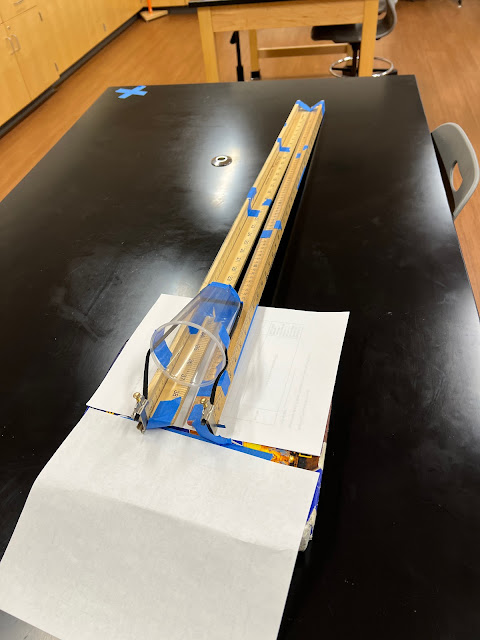## Wednesday, October 5, 2022

### Applied Concepts: Elastic Thing Launcher

Where are we going with this? The information on this page connects projectile motion with conservation of energy where in potential energy is converted to how far a projectile travels.

Applied Concepts: Elastic Thing Launcher
(Okay, this should be interesting!)

Projectile motion is a fundamental aspect of the study of motion in physics. There are many ways to impart velocity at some angle to a projectile.

One way is to attach a "pusher" to some sort of spring or elastic band. Pull it back… BAM!

Think of a slingshot… Now, let the cup ride on rails…The projectile, a squishy ball, rides in the cup that is pulled backand released.

Four quantities are easily measured in this apparatus: The mass of the projectile, the angle of the "thrower," how far back the elastic band was stretched, and how far (distance) that the projectile travels.

From those, everything else can be calculated! Really…

An interesting exercise would be to reverse math back to the coefficient of elasticity in the elastic band (or spring).

Says who?

Stretching the elastic band stores potential energy in the band. And, of course, there is a formula for that!

PE = 1/2kd2

where PE is elastic potential energy, k is the elastic constant, a function of the material, and d is the distance that the spring / band is stretched.

Often you will see this as

PE = 1/2k∆d2

where ∆d is the stretch. Of course, other notations exist! Of course.

Okay… so… When you release the band, most of the energy stored is converted to kinetic energy. While some of that energy will be in the "pusher" (cup in image above) if the mass of the cup is small compared to the projectile, then it's probably okay to ignore it. An even smaller amount will go into friction as the cup rubs on the rails.

Suppose the mass of the "pusher" is 7 grams and the mass of the projectile is 14 grams… Hmm… I'm betting someone weighed something! Then we can say that 2/3 of the potential energy goes into the projectile and 1/3 goes into the "pusher."

So, what now?

Measuring the angle of the rails and the distance that the projectile travels leads to being able to find the initial velocity of the projectile:

_____________

SUMMARY

So, if you are given theta and the velocity, here's a checklist sort of process…
• #1 Draw the diagram and label everything.

• #2 Find the component velocities in the x and y directions:
v • cosθ = vx
v • sinθ = vy

• #3 Find the time up using vy and acceleration due to gravity (probably 9.81 m/s/s)
tup = v / 9.81

• #4 Find the total time where…
t = tup + tdown

and

tup = tdown

so

t = 2•tup

• #5 Use the distance equation to find the horizontal displacement:

d= vx•t
where vx was found in step 2 and was found in step 4.

• #6 BONUS: Find the maximum vertical displacement:

dy = vytup + 1/2atup2
where a = -9.81 m/s/s,  vy was found in step 2 and tup was found in step 3.

_____________
GIANT MESS But actually COOL

d= v• t
dv • cosθ • t
dv • cosθ • 2 • tup
dv • cosθ • 2 • v / 9.81
dv • cosθ • 2 • v • sinθ / 9.81
d= 2 • v2 • cosθ • sinθ / 9.81
wait for the magic!
d= 2 • v2 • 1/2 • sin2θ / 9.81
d=  v2 • sin2θ / 9.81

Say you wanted to find v for some distance x?

9.81 • d=  v2 • sin2θ

(9.81 • d) / sin2θ =  v2

√ (9.81 • dx ) / sin2θ   = v

_____________

Okay, so… wow! Is this why we take math classes?

Thus, from the distance and and angle we get the velocity.

And since the potential energy was converted to kinetic energy…

Whereas

EK = 1/2mv2

and whereas

EK ≈ EP

We can backtrack to find the elasticity.

Considering the materials used, we earlier decided that 2/3 of the potential energy was transferred to the projectile. Thus, in this specific case, and recalling that

PE = 1/2kd2

EK = 2/3 EP

We can state that:

1/2mv2 = 2/3 (1/2kd2)

Thus, after finding v, solving for k becomes an exercise easily completed by the reader.